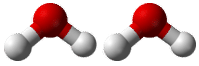# 5.21: Conversions Between Moles and Atoms

$$\newcommand{\vecs}{\overset { \rightharpoonup} {\mathbf{#1}} }$$ $$\newcommand{\vecd}{\overset{-\!-\!\rightharpoonup}{\vphantom{a}\smash {#1}}}$$$$\newcommand{\id}{\mathrm{id}}$$ $$\newcommand{\Span}{\mathrm{span}}$$ $$\newcommand{\kernel}{\mathrm{null}\,}$$ $$\newcommand{\range}{\mathrm{range}\,}$$ $$\newcommand{\RealPart}{\mathrm{Re}}$$ $$\newcommand{\ImaginaryPart}{\mathrm{Im}}$$ $$\newcommand{\Argument}{\mathrm{Arg}}$$ $$\newcommand{\norm}{\| #1 \|}$$ $$\newcommand{\inner}{\langle #1, #2 \rangle}$$ $$\newcommand{\Span}{\mathrm{span}}$$ $$\newcommand{\id}{\mathrm{id}}$$ $$\newcommand{\Span}{\mathrm{span}}$$ $$\newcommand{\kernel}{\mathrm{null}\,}$$ $$\newcommand{\range}{\mathrm{range}\,}$$ $$\newcommand{\RealPart}{\mathrm{Re}}$$ $$\newcommand{\ImaginaryPart}{\mathrm{Im}}$$ $$\newcommand{\Argument}{\mathrm{Arg}}$$ $$\newcommand{\norm}{\| #1 \|}$$ $$\newcommand{\inner}{\langle #1, #2 \rangle}$$ $$\newcommand{\Span}{\mathrm{span}}$$$$\newcommand{\AA}{\unicode[.8,0]{x212B}}$$Figure $$\PageIndex{1}$$ (Credit: Courtesy of Diane A. Reid/National Cancer Institute; Source: http://commons.wikimedia.org/wiki/File:Man_responds_to_telephone_call.jpg(opens in new window); License: Public Domain)

## Big numbers or little numbers?

Do you hate to type subscripts and superscripts? Even with a good word-processing program, having to click on an icon to get a superscript and then remembering to click off after you type the number can be a real hassle. If we did not know about moles and just knew about numbers of atoms or molecules (those big numbers that require lots of superscripts), life would be much more complicated and we would make many more typing errors.

## Conversions Between Moles and Atoms

### Conversions Between Moles and Number of Particles

Using our unit conversion techniques, we can use the mole label to convert back and forth between the number of particles and moles.

##### Example $$\PageIndex{1}$$: Converting Number of Particles to Moles

The element carbon exists in two primary forms: graphite and diamond. How many moles of carbon atoms is $$4.72 \times 10^{24}$$ atoms of carbon?

###### Known
• number of $$\ce{C}$$ atoms $$= 4.72 \times 10^{24}$$
• $$1$$ mole $$= 6.02 \times 10^{23}$$ atoms
###### Unknown
• 4.72 x 1024 = ? mol C

One conversion factor will allow us to convert from the number of $$\ce{C}$$ atoms to moles of $$\ce{C}$$ atoms.

###### Step 2: Calculate.

$4.72 \times 10^{24} \: \text{atoms} \: \ce{C} \times \frac{1 \: \text{mol} \: \ce{C}}{6.02 \times 10^{23} \: \text{atoms} \: \ce{C}} = 7.84 \: \text{mol} \: \ce{C}\nonumber$

The given number of carbon atoms was greater than Avogadro's number, so the number of moles of $$\ce{C}$$ atoms is greater than 1 mole. Since Avogadro's number is a measured quantity with three significant figures, the result of the calculation is rounded to three significant figures.

Suppose that you want to know how many hydrogen atoms are in a mole of water molecules. First, you need to know the chemical formula for water, which is $$\ce{H_2O}$$. There are two atoms of hydrogen in each molecule of water. How many atoms of hydrogen are in two water molecules? There are $$2 \times 2 = 4$$ hydrogen atoms. How about in a dozen? In that case, a dozen is 12; so $$12 \times 2 = 24$$ hydrogen atoms in a dozen water molecules. To get the answers (4 and 24), you multiply the given number of molecules by two atoms of hydrogen per molecule. So, to find the number of hydrogen atoms in a mole of water molecules, the problem can be solved using conversion factors:

$1 \: \text{mol} \: \ce{H_2O} \times \frac{6.02 \times 10^{23} \: \text{molecules} \: \ce{H_2O}}{1 \: \text{mol} \: \ce{H_2O}} \times \frac{2 \: \text{atoms} \: \ce{H}}{1 \: \text{molecule} \: \ce{H_2O}} = 1.20 \times 10^{24} \: \text{atoms} \: \ce{H}\nonumber$

The first conversion factor converts from moles of particles to the number of particles. The second conversion factor reflects the number of atoms contained within each molecule.Figure $$\PageIndex{2}$$: Two water molecules contain 4 hydrogen atoms and 2 oxygen atoms. A mole of water molecules contains 2 moles of hydrogen atoms and 1 mole of oxygen atoms. (Credit: Ben Mills (Wikimedia: Benjah-bmm27); Source: http://commons.wikimedia.org/wiki/File:Water-3D-balls-A.png(opens in new window); License: Public Domain)
##### Example $$\PageIndex{2}$$: Atoms, Molecules, and Moles

Sulfuric acid has the chemical formula $$\ce{H_2SO_4}$$. A certain quantity of sulfuric acid contains $$4.89 \times 10^{25}$$ atoms of oxygen. How many moles of sulfuric acid is the sample?

###### Known
• $$4.89 \times 10^{25} = \ce{O}$$ atoms
• $$1$$ mole $$= 6.02 \times 10^{23}$$ molecules $$\ce{H_2SO_4}$$
###### Unknown
• mol of H2SO4 molecules

Two conversion factors will be used. First, convert atoms of oxygen to molecules of sulfuric acid. Then, convert molecules of sulfuric acid to moles of sulfuric acid.

###### Step 2: Calculate.

$4.89 \times 10^{25} \: \text{atoms} \: \ce{O} \times \frac{1 \: \text{molecule} \: \ce{H_2SO_4}}{4 \: \text{atoms} \: \ce{O}} \times \frac{1 \: \text{mol} \: \ce{H_2SO_4}}{6.02 \times 10^{23} \: \text{molecules} \: \ce{H_2SO_4}} = 20.3 \: \text{mol} \: \ce{H_2SO_4}\nonumber$

The original number of oxygen atoms was about 80 times larger than Avogadro's number. Since each sulfuric acid molecule contains 4 oxygen atoms, there are about 20 moles of sulfuric acid molecules.

## Summary

• Methods are described for conversions between moles, atoms, and molecules.

## Review

1. What conversion factor would we need to convert moles of helium to atoms of helium?
2. I want to convert atoms to moles. My friend tells me to multiply the number of atoms by 6.02 × 1023 atoms/mole. Is my friend correct?
3. Why do you need to know the formula for a molecule in order to calculate the number of moles of one of the atoms?
4. How many atoms of fluorine are in 5.6×1022 molecules of MgF2?

5.21: Conversions Between Moles and Atoms is shared under a CC BY-NC license and was authored, remixed, and/or curated by LibreTexts.